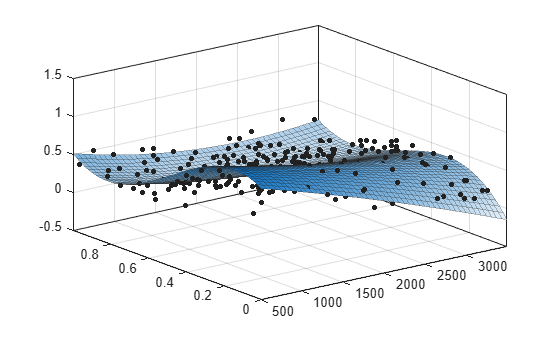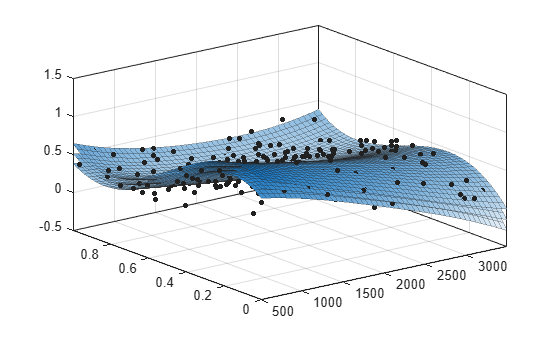# Evaluate Surface Fit

This example shows how to work with a surface fit.

### Load Data and Fit a Polynomial Surface

```load franke; surffit = fit([x,y],z,'poly23','normalize','on')```
``` Linear model Poly23: surffit(x,y) = p00 + p10*x + p01*y + p20*x^2 + p11*x*y + p02*y^2 + p21*x^2*y + p12*x*y^2 + p03*y^3 where x is normalized by mean 1982 and std 868.6 and where y is normalized by mean 0.4972 and std 0.2897 Coefficients (with 95% confidence bounds): p00 = 0.4253 (0.3928, 0.4578) p10 = -0.106 (-0.1322, -0.07974) p01 = -0.4299 (-0.4775, -0.3822) p20 = 0.02104 (0.001457, 0.04062) p11 = 0.07153 (0.05409, 0.08898) p02 = -0.03084 (-0.05039, -0.01129) p21 = 0.02091 (0.001372, 0.04044) p12 = -0.0321 (-0.05164, -0.01255) p03 = 0.1216 (0.09929, 0.1439) ```

The output displays the fitted model equation, the fitted coefficients, and the confidence bounds for the fitted coefficients.

### Plot the Fit, Data, Residuals, and Prediction Bounds

`plot(surffit,[x,y],z)`Plot the residuals fit.

`plot(surffit,[x,y],z,'Style','Residuals')`Plot prediction bounds on the fit.

`plot(surffit,[x,y],z,'Style','predfunc')`### Evaluate the Fit at a Specified Point

Evaluate the fit at a specific point by specifying a value for `x` and `y` , using this form: `z = fittedmodel(x,y)`.

`surffit(1000,0.5)`
```ans = 0.5673 ```

### Evaluate the Fit Values at Many Points

```xi = [500;1000;1200]; yi = [0.7;0.6;0.5]; surffit(xi,yi)```
```ans = 3×1 0.3771 0.4064 0.5331 ```

Get prediction bounds on those values.

`[ci, zi] = predint(surffit,[xi,yi])`
```ci = 3×2 0.0713 0.6829 0.1058 0.7069 0.2333 0.8330 ```
```zi = 3×1 0.3771 0.4064 0.5331 ```

### Get the Model Equation

Enter the fit name to display the model equation, fitted coefficients, and confidence bounds for the fitted coefficients.

`surffit`
``` Linear model Poly23: surffit(x,y) = p00 + p10*x + p01*y + p20*x^2 + p11*x*y + p02*y^2 + p21*x^2*y + p12*x*y^2 + p03*y^3 where x is normalized by mean 1982 and std 868.6 and where y is normalized by mean 0.4972 and std 0.2897 Coefficients (with 95% confidence bounds): p00 = 0.4253 (0.3928, 0.4578) p10 = -0.106 (-0.1322, -0.07974) p01 = -0.4299 (-0.4775, -0.3822) p20 = 0.02104 (0.001457, 0.04062) p11 = 0.07153 (0.05409, 0.08898) p02 = -0.03084 (-0.05039, -0.01129) p21 = 0.02091 (0.001372, 0.04044) p12 = -0.0321 (-0.05164, -0.01255) p03 = 0.1216 (0.09929, 0.1439) ```

To get only the model equation, use `formula`.

`formula(surffit)`
```ans = 'p00 + p10*x + p01*y + p20*x^2 + p11*x*y + p02*y^2 + p21*x^2*y + p12*x*y^2 + p03*y^3' ```

### Get Coefficient Names and Values

Specify a coefficient by name.

`p00 = surffit.p00`
```p00 = 0.4253 ```
`p03 = surffit.p03`
```p03 = 0.1216 ```

Get all the coefficient names. Look at the fit equation (for example, `f(x,y) = p00 + p10*x...`) to see the model terms for each coefficient.

`coeffnames(surffit)`
```ans = 9x1 cell {'p00'} {'p10'} {'p01'} {'p20'} {'p11'} {'p02'} {'p21'} {'p12'} {'p03'} ```

Get all the coefficient values.

`coeffvalues(surffit)`
```ans = 1×9 0.4253 -0.1060 -0.4299 0.0210 0.0715 -0.0308 0.0209 -0.0321 0.1216 ```

### Get Confidence Bounds on the Coefficients

Use confidence bounds on coefficients to help you evaluate and compare fits. The confidence bounds on the coefficients determine their accuracy. Bounds that are far apart indicate uncertainty. If the bounds cross zero for linear coefficients, this means you cannot be sure that these coefficients differ from zero. If some model terms have coefficients of zero, then they are not helping with the fit.

`confint(surffit)`
```ans = 2×9 0.3928 -0.1322 -0.4775 0.0015 0.0541 -0.0504 0.0014 -0.0516 0.0993 0.4578 -0.0797 -0.3822 0.0406 0.0890 -0.0113 0.0404 -0.0126 0.1439 ```

### Find Methods

List every method that you can use with the fit.

`methods(surffit)`
```Methods for class sfit: argnames category coeffnames coeffvalues confint dependnames differentiate feval fitoptions formula indepnames islinear numargs numcoeffs plot predint probnames probvalues quad2d setoptions sfit type ```

For more information on how to use a fit method, see `sfit`.